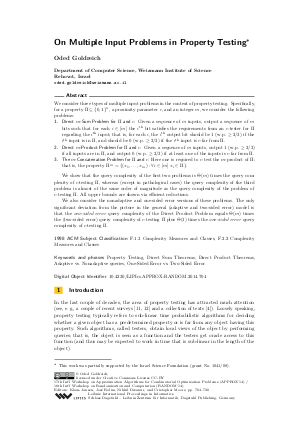Document# On Multiple Input Problems in Property Testing

### Author Oded Goldreich## File

LIPIcs.APPROX-RANDOM.2014.704.pdf
• Filesize: 0.52 MB
• 17 pages

## Cite As

Oded Goldreich. On Multiple Input Problems in Property Testing. In Approximation, Randomization, and Combinatorial Optimization. Algorithms and Techniques (APPROX/RANDOM 2014). Leibniz International Proceedings in Informatics (LIPIcs), Volume 28, pp. 704-720, Schloss Dagstuhl - Leibniz-Zentrum für Informatik (2014)
https://doi.org/10.4230/LIPIcs.APPROX-RANDOM.2014.704

## Abstract

We consider three types of multiple input problems in the context of property testing. Specifically, for a property Pi (of n-bit long strings), a proximity parameter epsilon, and an integer m, we consider the following problems: (1) Direct m-Sum Problem for Pi and epsilon: Given a sequence of m inputs, output a sequence of m bits such that for each i in [m] the i-th bit satisfies the requirements from an epsilon-tester for Pi regarding the i-th input; that is, for each i, the i-th output bit should be 1 (w.p. at least 2/3) if the i-th input is in Pi, and should be 0 (w.p. at least 2/3) if the i-th input is epsilon-far from Pi. (2) Direct m-Product Problem for Pi and epsilon: Given a sequence of m inputs, output 1 (w.p. at least 2/3) if all inputs are in Pi, and output 0 (w.p. at least 2/3) if at least one of the inputs is epsilon-far from Pi. (3) The m-Concatenation Problem for Pi and epsilon: Here one is required to epsilon-test the m-product of Pi; that is, the property that consists of the m-wise Cartesian product of Pi. We show that the query complexity of the first two problems is Theta(m) times the query complexity of epsilon-testing Pi, whereas (except in pathological cases) the query complexity of the third problem is almost of the same order of magnitude as the query complexity of the problem of epsilon-testing Pi. All upper bounds are shown via efficient reductions. We also consider the nonadaptive and one-sided error versions of these problems. The only significant deviation from the picture in the general (adaptive and two-sided error) model is that the one-sided error query complexity of the Direct Product Problem equals Theta(m) times the (two-sided error) query complexity of epsilon-testing Pi plus Theta(1) times the one-sided error query complexity of epsilon-testing Pi.
##### Keywords
• Property Testing
• Direct Sum Theorems
• Direct Product Theorems
• One-Sided Error vs Two-Sided Error

## Metrics

• Access Statistics
• Total Accesses (updated on a weekly basis)
0